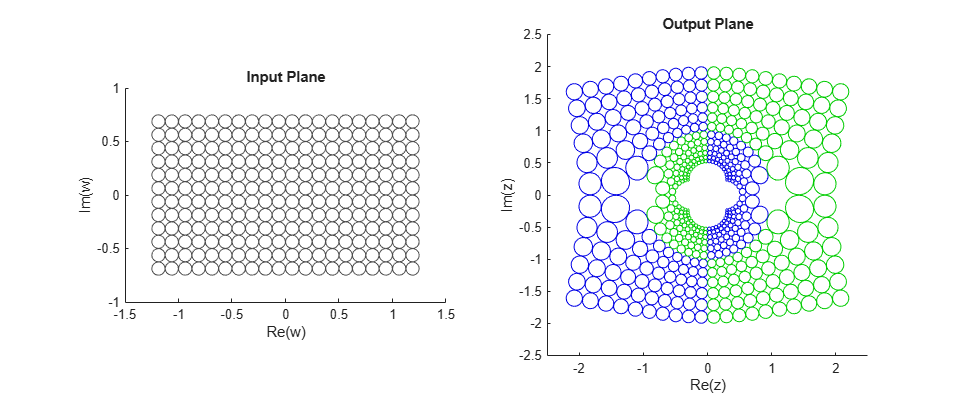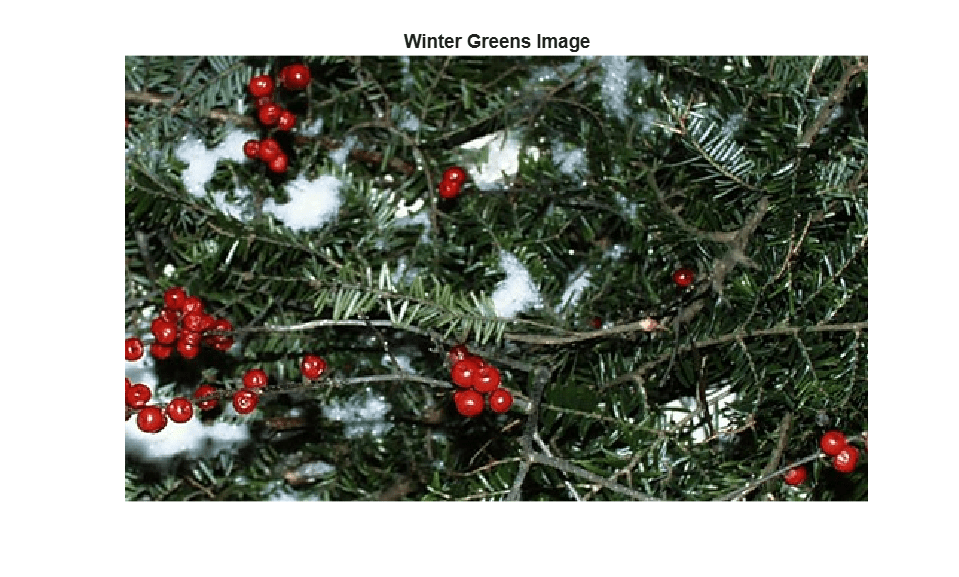Documentation

## Exploring a Conformal Mapping

This example shows how to explore a conformal mapping. Geometric image transformations are useful in understanding a conformal mapping that is important in fluid-flow problems, and the mapping itself can be used to transform imagery for an interesting special effect.

### Step 1: Select a Conformal Transformation

Conformal transformations, or mappings, have many important properties and uses. One property relevant to image transformation is the preservation of local shape (except sometimes at isolated points).

This example uses a 2-D conformal transformation to warp an image. The mapping from output to input, `g: R^2 -> R^2`, is defined in terms of a complex analytic function `G: C -> C`, where

` G(z) = (z + 1/z) / 2.`

We define `g` via a direct correspondence between each point `(x,y)` in `R^2` (the Euclidean plane) and the point `z = x + i*y` in `C` (the complex plane),

` g(x,y) = (Re(w),Im(w)) = (u,v)`

where

` w = u + i*v = G(x + i*y).`

This conformal mapping is important in fluid mechanics because it transforms lines of flow around a circular disk (or cylinder, if we add a third dimension) to straight lines. (See pp. 340-341 in Strang, Gilbert, Introduction to Applied Mathematics, Wellesley-Cambridge Press, Wellesley, MA, 1986.)

A note on the value of complex variables: although we could express the definition of `g` directly in terms of `x` and `y`, that would obscure the underlying simplicity of the transformation. This disadvantage would come back to haunt us in Step 3 below. There, if we worked purely in real variables, we would need to solve a pair of simultaneous nonlinear equations instead of merely applying the quadratic formula!

### Step 2: Warp an Image Using the Conformal Transformation

We start by loading the peppers image, extracting a 300-by-500 subimage, and displaying it.

``` A = imread('peppers.png'); A = A(31:330,1:500,:); figure imshow(A) title('Original Image','FontSize',14) ```Then use `maketform` to make a custom `tform` struct with a handle to function `conformalInverse` as its `INVERSE_FCN` argument:

```conformal = maketform('custom', 2, 2, [], @conformalInverse, []); ```

To view `conformalInverse` use:

```type conformalInverse.m ```
```function U = conformalInverse(X, ~) % conformalInverse Inverse conformal transformation. % % Supports conformal transformation example, ConformalMappingImageExample.m % ("Exploring a Conformal Mapping"). % Copyright 2005-2013 The MathWorks, Inc. Z = complex(X(:,1),X(:,2)); W = (Z + 1./Z)/2; U(:,2) = imag(W); U(:,1) = real(W); ```

Horizontal and vertical bounds are needed for mapping the original and transformed images to the input and output complex planes. Note that the proportions in `uData` and `vData` match the height-to-width ratio of the original image (3/5).

```uData = [ -1.25 1.25]; % Bounds for REAL(w) vData = [ 0.75 -0.75]; % Bounds for IMAG(w) xData = [ -2.4 2.4 ]; % Bounds for REAL(z) yData = [ 2.0 -2.0 ]; % Bounds for IMAG(z) ```

We apply `imtransform` using the `SIZE` parameter to ensure an aspect ratio that matches the proportions in `xData` and `yData` (6/5), and view the result.

```B = imtransform( A, conformal, 'cubic', ... 'UData', uData,'VData', vData,... 'XData', xData,'YData', yData,... 'Size', [300 360], 'FillValues', 255 ); figure imshow(B) title('Transformed Image','FontSize',14) ```Compare the original and transformed images. Except that the edges are now curved, the outer boundary of the image is preserved by the transformation. Note that each feature from the original image appears twice in the transformed image (look at the various peppers). And there is a hole in the middle of the transformed image with four regular cusps around its edges.

In fact, every point in the input w-plane is mapped to two points in the output `z`-plane, one inside the unit circle and one outside. The copies inside the unit circle are much smaller than those outside. It's clear that the cusps around the central hole are just the copies of the four image corners that mapped inside the unit circle.

### Step 3: Construct Forward Transformations

If the transformation created with `maketform` has a forward function, then we can apply `tformfwd` to regular geometric objects (in particular, to rectangular grids and uniform arrays of circles) to obtain further insight into the transformation. In this example, because `G` maps two output points to each input point, there is no unique forward transformation. But we can proceed if we are careful and work with two different forward functions.

Letting `w = (z + 1/z)/2` and solving the quadratic equation that results,

```z^2 + 2*w*z + 1 = 0, ```

we find that

```z = w +/- sqrt{(w^2 - 1). ```

The positive and the negative square roots lead to two separate forward transformations. We construct the first using `maketform` and a handle to the function, `conformalForward1`.

```t1 = maketform('custom', 2, 2, @conformalForward1, [], []); ```

To view `conformalForward1` use:

```type conformalForward1.m ```
```function X = conformalForward1(U, ~) % conformalForward1 Forward transformation with positive square root. % % Supports conformal transformation example, ConformalMappingImageExample.m % ("Exploring a Conformal Mapping"). % Copyright 2005-2013 The MathWorks, Inc. W = complex(U(:,1),U(:,2)); Z = W + sqrt(W.^2 - 1); X(:,2) = imag(Z); X(:,1) = real(Z); ```

We construct the second transformation with another function that is identical to `conformalForward1` except for a sign change.

```t2 = maketform('custom', 2, 2, @conformalForward2, [], []); type conformalForward2.m ```
```function X = conformalForward2(U, ~) % conformalForward2 Forward transformation with negative square root. % % Supports conformal transformation example, ConformalMappingImageExample.m % ("Exploring a Conformal Mapping"). % Copyright 2005-2013 The MathWorks, Inc. W = complex(U(:,1),U(:,2)); Z = W - sqrt(W.^2 - 1); X(:,2) = imag(Z); X(:,1) = real(Z); ```

### Step 4: Explore the Mapping Using Grid Lines

With the two forward transformations, we can illustrate the mapping of a grid of lines, using additional helper functions.

```f3 = figure('Name','Conformal Transformation: Grid Lines'); axIn = conformalSetupInputAxes( subplot(1,2,1)); axOut = conformalSetupOutputAxes(subplot(1,2,2)); conformalShowLines(axIn, axOut, t1, t2) % Reduce wasted vertical space in figure f3.Position = [1 1 1 0.7] .* f3.Position; ```You can see that the grid lines are color-coded according to their quadrants in the input plane before and after the transformations. The colors also follow the transformed grids to the output planes. Note that each quadrant transforms to a region outside the unit circle and to a region inside the unit circle. The right-angle intersections between grid lines are preserved under the transformation -- evidence of the shape-preserving property of conformal mappings -- except for the points at +1 and -1 on the real axis.

### Step 5: Explore the Mapping Using Packed Circles

Under a conformal transformation, small circles should remain nearly circular, changing only in position and size. Again applying the two forward transformations, this time we map a regular array of uniformly-sized circles.

```f4 = figure('Name','Conformal Transformation: Circles'); axIn = conformalSetupInputAxes( subplot(1,2,1)); axOut = conformalSetupOutputAxes(subplot(1,2,2)); conformalShowCircles(axIn, axOut, t1, t2) % Reduce wasted vertical space in figure f4.Position = [1 1 1 0.7] .* f4.Position; ```You can see that the transform to a circle packing where tangencies have been preserved. In this example, the color coding indicates use of the positive (green) or negative (blue) square root of `w^2 - 1`. Note that the circles change dramatically but that they remain circles (shape-preservation, once again).

### Step 6: Explore the Mapping Using Images

To further explore the conformal mapping, we can place the input and transformed images on the pair of axes used in the preceding examples and superpose a set of curves as well.

First we display the input image, rendered semi-transparently, over the input axes of the conformal map, along with a black ellipse and a red line along the real axis.

```figure axIn = conformalSetupInputAxes(axes); conformalShowInput(axIn, A, uData, vData) title('Original Image Superposed on Input Plane','FontSize',14) ```Next we display the output image over the output axes of the conformal map, along with two black circles and one red circle. Again, the image is semi-transparent.

```figure axOut = conformalSetupOutputAxes(axes); conformalShowOutput(axOut, B, xData, yData) title('Transformed Image Superposed on Output Plane','FontSize',14) ```MATLAB® graphics made it easy to shift and scale the original and transformed images to superpose them on the input (`w-`) and output (`z-`) planes, respectively. The use of semi-transparency makes it easier to see the ellipse, line, and circles. The ellipse in the w-plane has intercepts at 5/4 and -5/4 on the horizontal axis and 3/4 and -3/4 on the vertical axis. `G` maps two circles centered on the origin to this ellipse: the one with radius 2 and the one with radius 1/2. And, as shown in red, `G` maps the unit circle to the interval [-1 1] on the real axis.

### Step 7: Obtain a Special Effect by Masking Parts of the Output Image

If the inverse transform function within a custom `tform` struct returns a vector filled with `NaN` for a given output image location, then `imtransform` (and also `tformarray`) assign the specified fill value at that location. In this step we repeat Step 1, but modify our inverse transformation function slightly to take advantage of this feature.

```type conformalInverseClip.m ```
```function U = conformalInverseClip( X, ~) % conformalInverseClip Inverse conformal transformation with clipping. % % This is a modification of conformalInverse in which points in X % inside the circle of radius 1/2 or outside the circle of radius 2 map to % NaN + i*NaN. % % Supports conformal transformation example, ConformalMappingImageExample.m % ("Exploring a Conformal Mapping"). % Copyright 2000-2013 The MathWorks, Inc. Z = complex(X(:,1),X(:,2)); W = (Z + 1./Z)/2; q = 0.5 <= abs(Z) & abs(Z) <= 2; W(~q) = complex(NaN,NaN); U(:,2) = imag(W); U(:,1) = real(W); ```

This is the same as the function defined in Step 2, except for the two additional lines:

```q = 0.5 <= abs(Z) & abs(Z) <= 2; W(~q) = complex(NaN,NaN);```

which cause the inverse transformation to return `NaN` at any point not between the two circles with radii of 1/2 and 2, centered on the origin. The result is to mask that portion of the output image with the specified fill value.

```ring = maketform('custom', 2, 2, [], @conformalInverseClip, []); Bring = imtransform( A, ring, 'cubic',... 'UData', uData, 'VData', vData,... 'XData', [-2 2], 'YData', yData,... 'Size', [400 400], 'FillValues', 255 ); figure imshow(Bring) title('Transformed Image With Masking','FontSize',14); ```The result is identical to our initial transformation except that the outer corners and inner cusps have been masked away to produce a ring effect.

### Step 8: Repeat the Effect on a Different Image

Applying the "ring" transformation to an image of winter greens (hemlock and alder berries) leads to an aesthetic special effect.

Load the image `greens.jpg`, which already has a 3/5 height-to-width ratio, and display it.

```C = imread('greens.jpg'); figure imshow(C) title('Winter Greens Image','FontSize',14); ```Transform the image and display the result, this time creating a square output image.

```D = imtransform( C, ring, 'cubic',... 'UData', uData, 'VData', vData,... 'XData', [-2 2], 'YData', [-2 2],... 'Size', [400 400], 'FillValues', 255 ); figure imshow(D) title('Transformed and Masked Winter Greens Image','FontSize',14); ```Notice that the local shapes of objects in the output image are preserved. The alder berries stayed round!